Chapter 12, Problem 45CR### Introductory Chemistry: A Foundati...

9th Edition
Steven S. Zumdahl + 1 other
ISBN: 9781337399425

#### Solutions

Chapter
Section### Introductory Chemistry: A Foundati...

9th Edition
Steven S. Zumdahl + 1 other
ISBN: 9781337399425
Textbook Problem
1 views

# Based on the electron configuration of the simple ions that the following pairs of elements would be expected to form, predict the formula of the simple binary compound that would be formed by each pair.msp;  a . Al and Cl         d . Ca and O b . Na and N        e . K and O c . Mg and S         f . sr and Br

Interpretation Introduction

(a)

Interpretation:

Predict the simple binary compound that can be formed by the use of electron configuration of the simple ions which are formed by those elements.

Concept Introduction:

Electron configuration describes the positions of electrons of an atom. Depending on that the periodic table is created. Periodic table further defines the simple ion that can be formed by any element. And using those ions, simple binary compounds can be produced.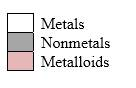Order in which orbitals fill to produce the atoms in periodic table as follows:

1s22s22p63s23p64s23d104p65s24d105p66s24f145d106p67s25f146d107p6

Simplest ion form by each group as follows: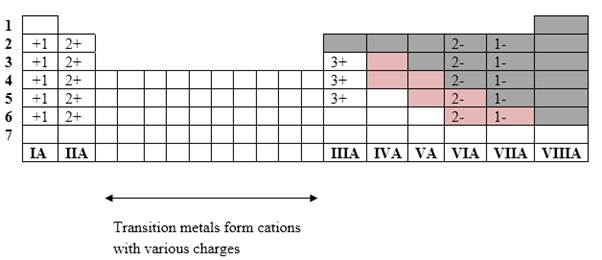If it’s a compound made by a combination of metal and nonmetal elements, called as ionic compounds.

Ionic chemical compound must have a zero charge. If a compound contains ions;

1. Both positive ions (cations) and negative ions (anions) must be there.

2. No: of cations and anions collectively should produce a zero-net charge.

Explanation

Al and Cl

Al, Z= 13  1s22s22p63s23p1     ( Group III A element ( Simple ion = Al3+

Cl, Z= 17  1s22s22p6</

Interpretation Introduction

(b)

Interpretation:

Predict the simple binary compound that can be formed by the use of electron configuration of the simple ions which are formed by those elements.

Concept Introduction:

Electron configuration describes the positions of electrons of an atom. Depending on that the periodic table is created. Periodic table further defines the simple ion that can be formed by any element. And using those ions, simple binary compounds can be produced.Order in which orbitals fill to produce the atoms in periodic table as follows:

1s22s22p63s23p64s23d104p65s24d105p66s24f145d106p67s25f146d107p6

Simplest ion form by each group as follows: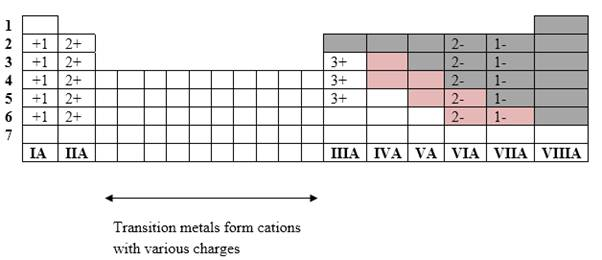If it’s a compound made by a combination of metal and nonmetal elements, called as ionic compounds.

Ionic chemical compound must have a zero charge. If a compound contains ions;

1. Both positive ions (cations) and negative ions (anions) must be there.

2. No: of cations and anions collectively should produce a zero-net charge.

Interpretation Introduction

(c)

Interpretation:

Predict the simple binary compound that can be formed by the use of electron configuration of the simple ions which are formed by those elements.

Concept Introduction:

Electron configuration describes the positions of electrons of an atom. Depending on that the periodic table is created. Periodic table further defines the simple ion that can be formed by any element. And using those ions, simple binary compounds can be produced.Order in which orbitals fill to produce the atoms in periodic table as follows:

1s22s22p63s23p64s23d104p65s24d105p66s24f145d106p67s25f146d107p6

Simplest ion form by each group as follows: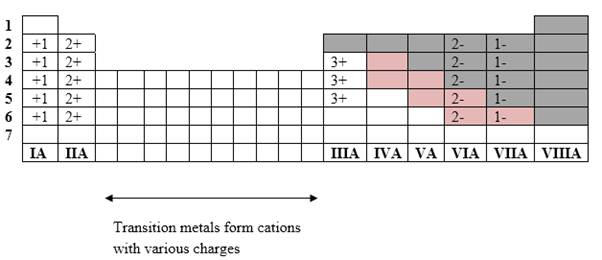If it’s a compound made by a combination of metal and nonmetal elements, called as ionic compounds.

Ionic chemical compound must have a zero charge. If a compound contains ions;

1. Both positive ions (cations) and negative ions (anions) must be there.

2. No: of cations and anions collectively should produce a zero-net charge.

Interpretation Introduction

(d)

Interpretation:

Predict the simple binary compound that can be formed by the use of electron configuration of the simple ions which are formed by those elements.

Concept Introduction:

Electron configuration describes the positions of electrons of an atom. Depending on that the periodic table is created. Periodic table further defines the simple ion that can be formed by any element. And using those ions, simple binary compounds can be produced.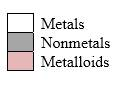Order in which orbitals fill to produce the atoms in periodic table as follows:

1s22s22p63s23p64s23d104p65s24d105p66s24f145d106p67s25f146d107p6

Simplest ion form by each group as follows: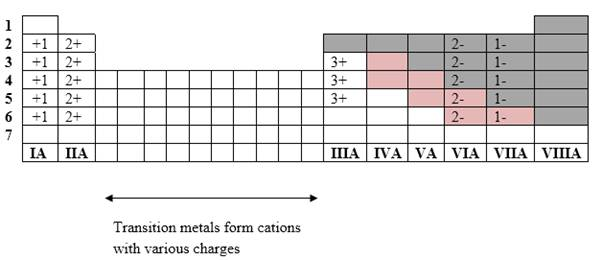If it’s a compound made by a combination of metal and nonmetal elements, called as ionic compounds.

Ionic chemical compound must have a zero charge. If a compound contains ions;

1. Both positive ions (cations) and negative ions (anions) must be there.

2. No: of cations and anions collectively should produce a zero-net charge.

Interpretation Introduction

(e)

Interpretation:

Predict the simple binary compound that can be formed by the use of electron configuration of the simple ions which are formed by those elements.

Concept Introduction:

Electron configuration describes the positions of electrons of an atom. Depending on that the periodic table is created. Periodic table further defines the simple ion that can be formed by any element. And using those ions, simple binary compounds can be produced.Order in which orbitals fill to produce the atoms in periodic table as follows:

1s22s22p63s23p64s23d104p65s24d105p66s24f145d106p67s25f146d107p6

Simplest ion form by each group as follows:If it’s a compound made by a combination of metal and nonmetal elements, called as ionic compounds.

Ionic chemical compound must have a zero charge. If a compound contains ions;

1. Both positive ions (cations) and negative ions (anions) must be there.

2. No: of cations and anions collectively should produce a zero-net charge.

Interpretation Introduction

(f)

Interpretation:

Predict the simple binary compound that can be formed by the use of electron configuration of the simple ions which are formed by those elements.

Concept Introduction:

Electron configuration describes the positions of electrons of an atom. Depending on that the periodic table is created. Periodic table further defines the simple ion that can be formed by any element. And using those ions, simple binary compounds can be produced.Order in which orbitals fill to produce the atoms in periodic table as follows:

1s22s22p63s23p64s23d104p65s24d105p66s24f145d106p67s25f146d107p6

Simplest ion form by each group as follows: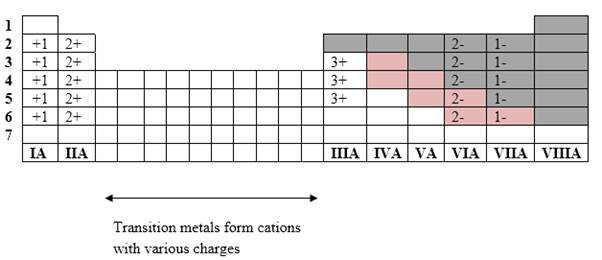If it’s a compound made by a combination of metal and nonmetal elements, called as ionic compounds.

Ionic chemical compound must have a zero charge. If a compound contains ions;

1. Both positive ions (cations) and negative ions (anions) must be there.

2. No: of cations and anions collectively should produce a zero-net charge.

### Still sussing out bartleby?

Check out a sample textbook solution.

See a sample solution

#### The Solution to Your Study Problems

Bartleby provides explanations to thousands of textbook problems written by our experts, many with advanced degrees!

Get Started

#### How do eating disorders affect health?

Understanding Nutrition (MindTap Course List)

#### Methylmercuryis a toxic industrial pollutant that is easily destroyed by cooking. T F

Nutrition: Concepts and Controversies - Standalone book (MindTap Course List)

#### What are sister chromatids?

Human Heredity: Principles and Issues (MindTap Course List)